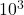## PLEASE HELP! The specific heat of octane, C8H18(l), is 2.22 J/(g⋅K). How much heat is needed to raise the temperature of 76.0 g

Question

The specific heat of octane, C8H18(l), is 2.22 J/(g⋅K).
How much heat is needed to raise the temperature of 76.0 g of octane from 10.6 ∘C to 26.0 ∘C?
Express your answer in joules to three significant figures.

in progress 0
6 months 2021-07-31T04:07:00+00:00 2 Answers 256 views 0

## Answers ( )

Q = 2.60 •J

Explanation:

Our specific heat capacity equation is:

Q = mC∆T

Q is the energy in joules.

m is the mass of the substance.

∆T is the temperature chance.

Let’s plug in what we know.

• We have 76.0 g of octane
• The specific heat capacity of octane is 2.22 J/(g•K)
• The temperature increases from 10.6º to 26.0º (a 15.4º increase)

Q = 76.0(2.22)(15.4)

Multiply.

Q = 2598.288

We want three significant figures.

Q = 2.60 •or

Q = 2590 J

Hope this helps!